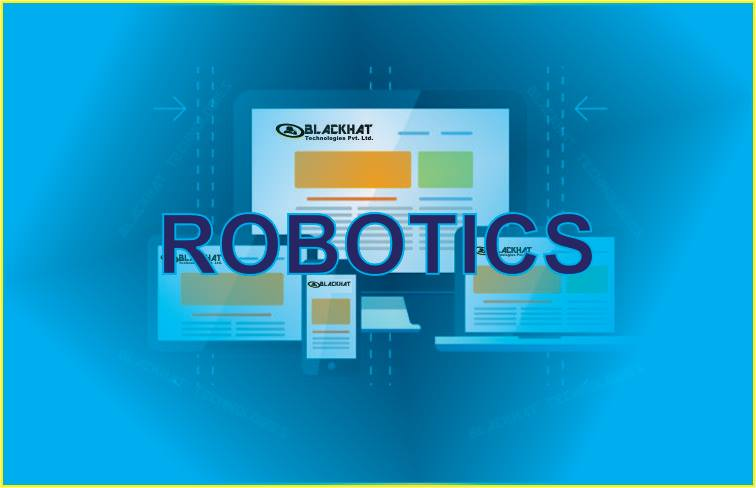Call Us on +91 7903891300Robotics Industrial Training In Patna

Course Fee: 4600/-@ 4000/- OnlyRobotics is concerned it is a branch of technology that deals with the design, construction, operation, structural disposition, manufacture and application of robots. Robotics is an exciting area of computer-controlled technology. Robotics is related to the sciences of electronics, engineering, mechanics, and software.

Robotics Engineering deals with the design, construction, operation, structural disposition, manufacture and application of robots and computer systems for their control, sensory feedback, and information processing. Program provides greater intelligence and higher versatility for robotic tasks under ever-changing constraints of the environment. Students are provided in-depth knowledge in the areas of Electro Mechanics, Robotics Sensors & Instrumentation, Robotics Fabrication, Artificial Intelligence and Robotic Vision. This builds a super specialization on fundamental background of core engineering.In today’s life the importance of robot is enhancing day by day in industrial life and other spheres.

Introduction Of Robotics

• History of Robots
• Types of Robots, Notation

Mathematical Representation of Robots

• Position and Orientation of a Rigid Body
• Some Properties of Rotation Matrices
• Successive Rotations,moving frame ZYZ
• Transformation between coordinate system
• Types of Joints: Rotary
• Representation of Links using Denvit - Hartenberg Parameters

Kinematics of Serial Manipulators

• Direct kinematics of 2R, 3R, RRP, RPR
• Puma560 manipulator
• SCARA manipulator
• Stanford Arm
• Inverse kinematics of 2R, 3R manipulator

Velocity and Statics of Manipulators

• Differential Relationships
• Jacobian
• Differential motions of a frame (translation and rotation)
• Linear and angular velocity of a rigid body
• links in serial manipulators, 2R, 3R manipulator
• Jacobian of serial manipulator
• Velocity ellipse of 2R manipulator
• Singularities of 2R manipulators
• Statics of serial manipulators
• Static force and torque analysis of 3R manipulator
• Singularity in force domain.

Dynamics of Manipulators

• Kinetic energy
• Potential energy
• Equation of motions
• Inertia of a link
• Recursive formulation of Dynamics using Newton Euler equation

Trajectory Planning

• Joint space schemes
• Cubic Trajectory
• Joint space schemes with via points
• Cubic trajectory with a via point
• Third order polynomial trajectory planning
• Linear segments with parabolic blends
• Cartesian space schemes
• Cartesian straight line and circular motion planning

Control

• Feedback control of a single link manipulator- first order
• second order system, PID control
• PID control of multi link manipulator
• Force control of manipulator
• force control of single mass
• Partitioning a task for force and position control- lever
• peg in hole Hybrid force
• position controller

Actuators

• Types
• Characteristics of actuating system
• proportional feedback control
• Electric motors
• DC motors, Reversible AC motors, Brushles DC motors, Stepper motors
• stepper motor speed-torque characteristics

Sensors

• Sensor characteristics
• Position sensors- potentiometers
• Encoders, LVDT, Resolvers
• Displacement sensor
• Velocity sensor-encoders, tachometers
• Acceleration sensors
• Torque sensors
• Touch and tactile sensor
• Proximity sensors-magnetic
• optical, ultrasonic, inductive, capacitive, eddy-current proximity sensors